Mechanical Engineering Software - Math Software for Mechanical Engineers

# Math Software for Mechanical Engineers

From kinematics and dynamics to heat transfer and fluid flow, Maple delivers a robust, auditable environment for the types of analyses performed by Mechanical Engineers.

###Mechanical Engineers Need the Features You Get in Maple

Mechanical engineers are simply unique. Their work is technically challenging, broad in scope, and subject to tighter and tighter deadlines.
Maplesoft understands these challenges. We’ve built feature into Maple that specifically address them, such as:

A Maple document combines live math, text, images and plots in a single document. In effect, Maple captures the inherent assumptions and thought process behind an analysis, as well as the calculations.

## High-Level Symbolic and Numeric Math

Maple offers practical high-level tools for numeric and symbolic math, data analysis, and programming. These tools are designed for both simple and complex engineering problems.

The symbolic and numeric math engines are seamlessly connected; parameters, equations and calculations can fluidly flow between the two. This means you can derive and numerically evaluate your equations in a single cohesive workflow.

Moreover, Maple’s programming language benefits from an interactive development environment and can use any of Maple’s high-level math tools.

• Code is faster to develop, debug and verify
• Can use Maple’s high level math functions, and
• Is easier to read by humans

## Reduce Calculation Risk with Units

Nearly every single quantity a mechanical engineer encounters – whether it’s force, temperature or speed, has a unit. Units are fluidly integrated into Maple, and can be used in simple calculations as well as numeric equation solving, optimization and visualization.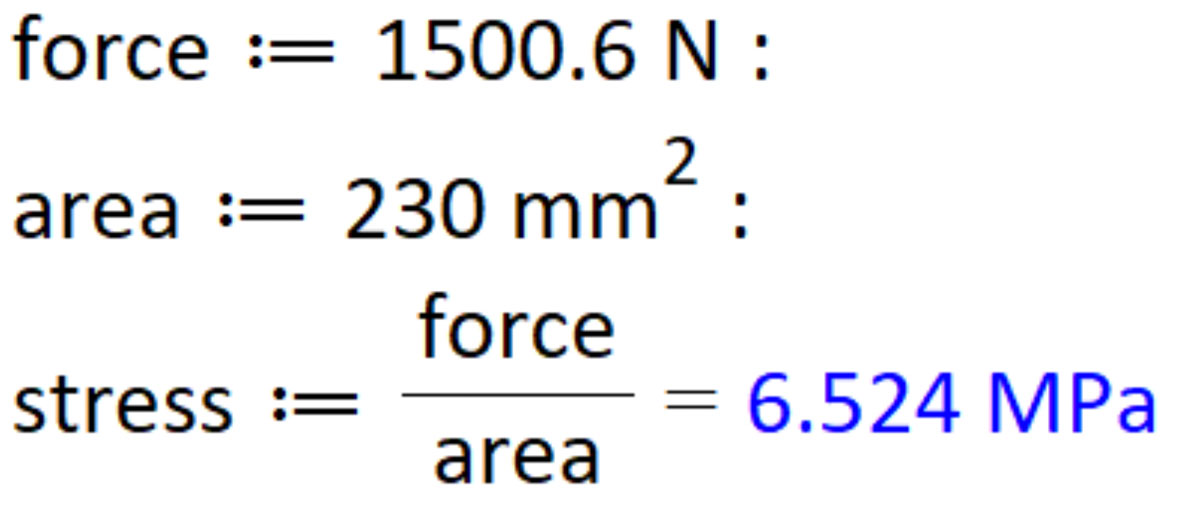Using units in calculations removes the risk of introducing unit conversion errors, and also acts as a check on the physical validity of the equations.

## Thermophysical and Thermochemical Data

Maple contains accurate, experimentally determined thermophysical and thermochemical data for pure fluids and fluid mixtures, and solids. Engineers use Maple to analyze refrigeration cycles, design heat exchangers, combustion and rocketry, psychrometric analysis and drying systems.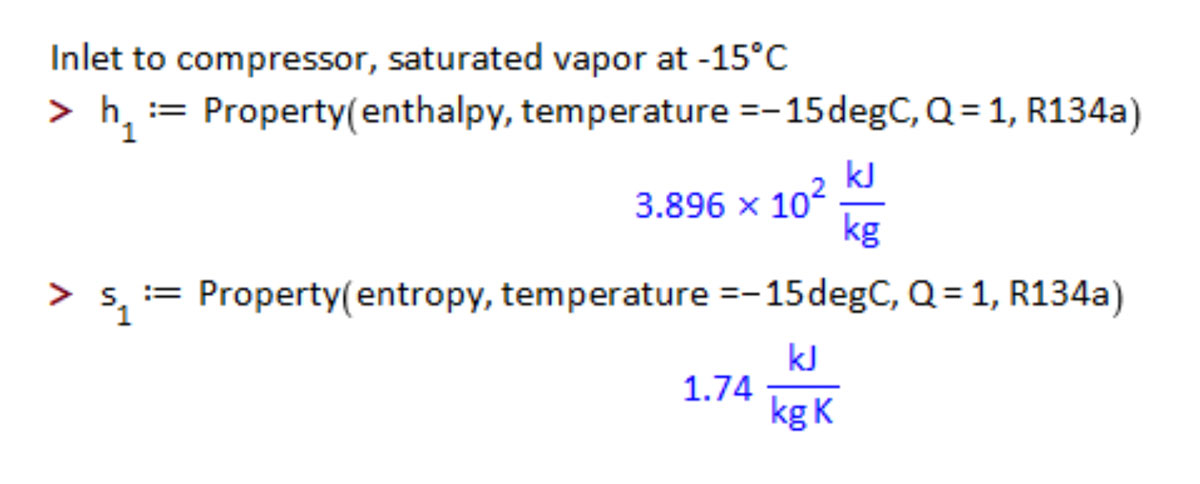###Mechanical Engineering Applications and User Stories

Given that Maple makes engineering math simple enough for a quick payoff, it’s not surprising that many mechanical engineers use Maple. Let’s now see what they do with Maple and learn about 5 applications of math software for Mechanical Engineers.

## Static Analysis of Trusses and Frames

The analyses of frames and trusses involve applying the concepts of static equilibrium to a system. The result is equations that describe the forces in a system.

The theoretical framework involves concepts such as the condition of static equilibrium (that is, the sum of the forces and moments at a point are zero), the method of joints and the method of sections

These concepts result in equations that have to be methodically derived and dimensionally balanced.

This requires natural math notation, rich documentation and the use of units. Units, in particular, act as a check on the physical validity of the equations.

Maple satisfies these requirements, with mathematically-live documents that can also feature images and text to assist in greater understanding.

These documents are auditable, and are a superior alternative to hand calculations on paper or spreadsheets.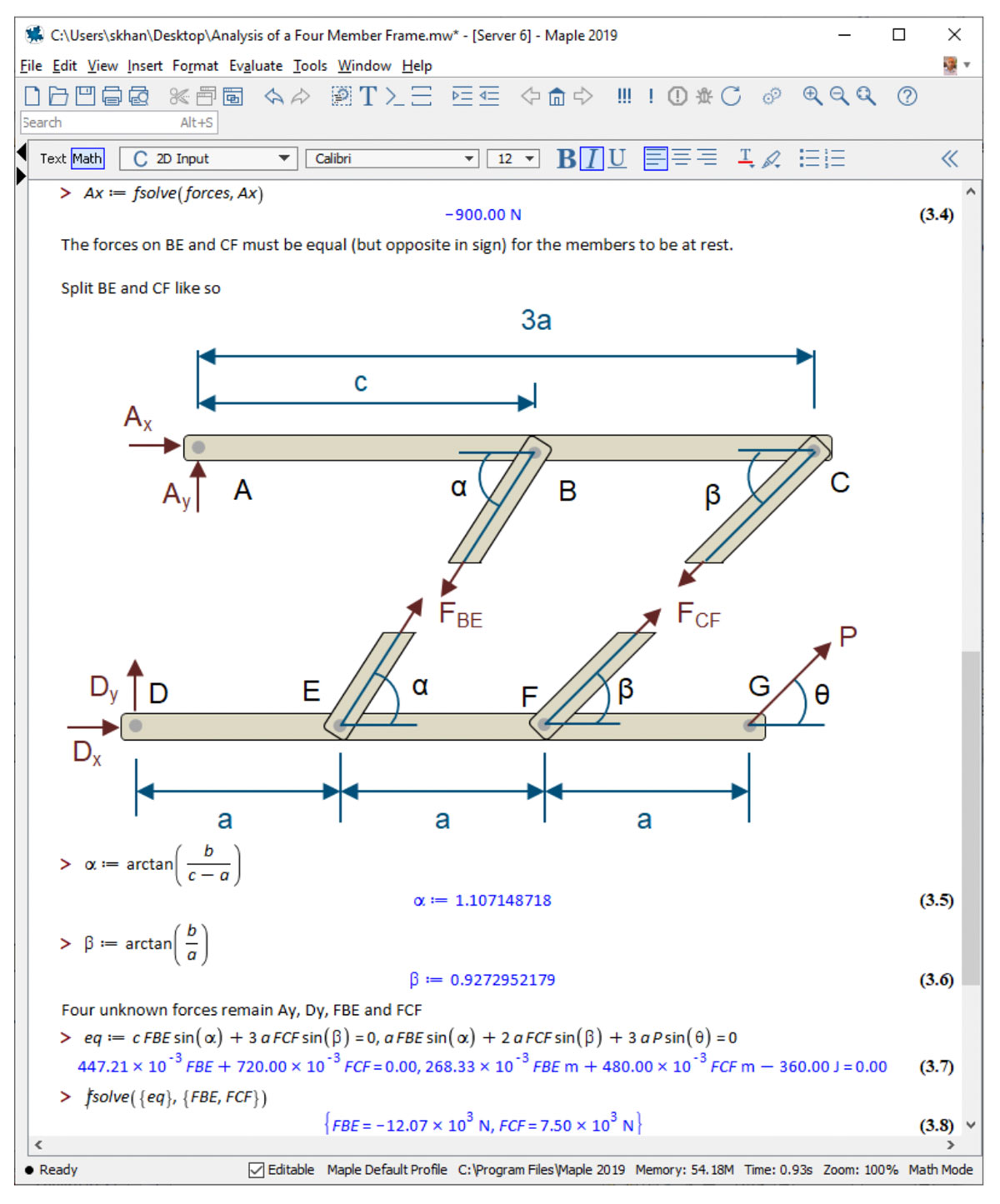## Kinematics of Mechanical Devices

Maple is the ideal environment for the equation-based kinematic analysis of mechanical devices.

• You can enter kinematic equations and geometric relationships, including differential equations, in auditable natural math notation.
• You can solve your kinematic equations using Maple’s powerful differential equation solvers or with matrix techniques
• Geometric equations can be rearranged and manipulated symbolically. Here, we differentiate the geometric constraints of a quick-return device to get the linear and angular velocity equations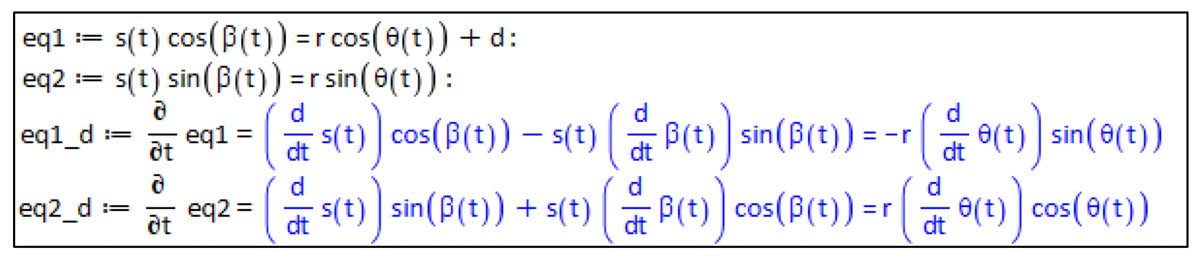• The motion of the device can be visualized and animated.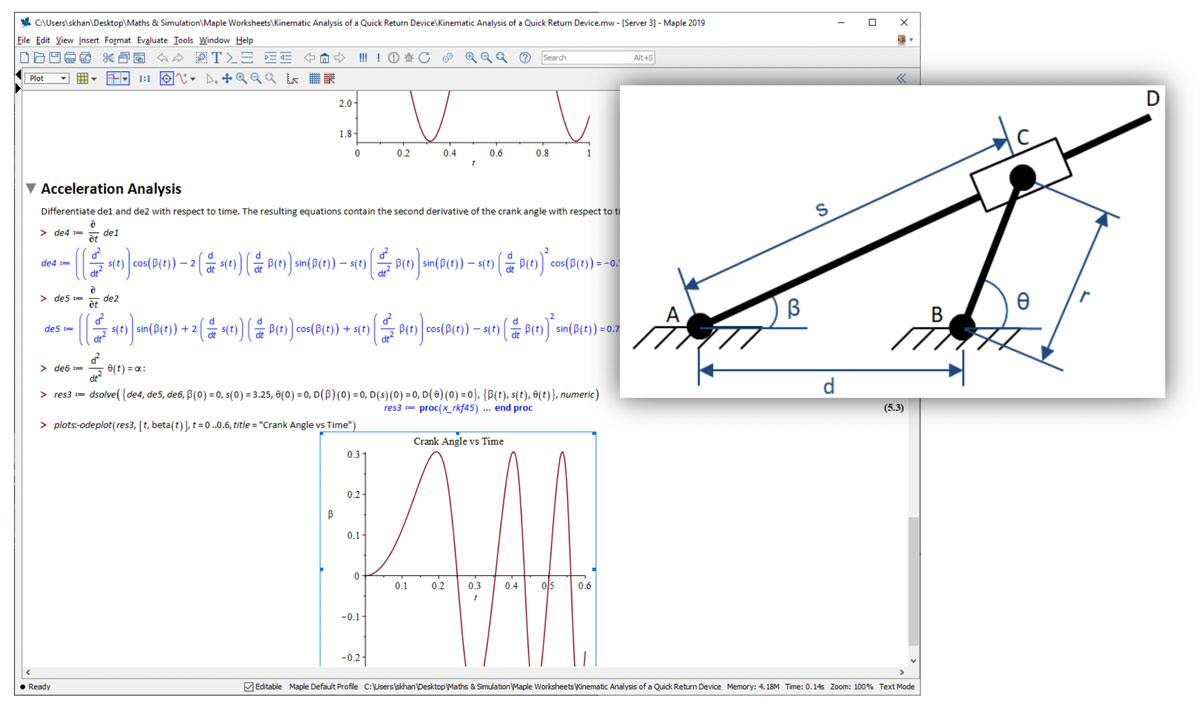## Heat Flows across a Thermodynamic Cycle

You can use Maple to calculate the heat flows around a thermodynamic cycle, such as vapor compression refrigeration cycles, Rankine cycles, Brayton cycles and more. You can even optimize the system to maximize the coefficient of performance.

Some users employ Maple to mode Organic Rankine Cycles – these are often used to recover low grade heat from, for example, factory exhausts.
Additionally, other mechanical engineers use Maple for steam turbine design.

These applications make use of Maple’s tools for thermodynamic and transport properties for fluids. This feature is units-aware – enthalpies, densities and other properties all have the appropriate units.This means you no longer have to rely on printed charts or lookup tables for fluid data.

With this feature supporting your work, you can calculate and optimize the heat flows around a thermodynamic cycle. Applications can also be deployed royalty-free with the Maple Player.## Fluid Flow Calculations

Mechanical engineers regularly solve fluid flow problems. They could be calculating pressure drops and flowrates in pipe networks, or examining the stresses and heat gains caused by compression of oil in a hydraulic cylinder.
These applications involve concepts such as Bernoulli’s Law, friction factors (for example, the Colebrook equation), and the transport properties of liquids.
These applications often require

• Natural math notation, to ensure equations can be fully validated
• Units, to eliminate conversion errors and ensure dimensional consistency
• Strong numerical solvers capable of resolving systems with parameters that vary greatly in magnitude
• Curve fitting, for fitting empirical data from pump data sheets to head curves## Robotics

Mechanical engineers are at the forefront of designing robotic systems, from the geometric and mechanical design to writing the controls and systems software.

Maple will help you develop the Denavit & Hartenberg transformation matrices that describe the motion of a multi-degree of freedom robot arm system. You can use then calculate the motion of each joint using Maple’s symbolic and numeric solvers. The symbolic solvers may even let you derive symbolic equations to describe the inverse kinematics of the system.If the problem admits such a solution, you can extract symbolic equations for each of the joint angles; this requires powerful symbolic solvers. Moreover, these equations can be translated into other programming languages, such as C, Java, Python, FORTRAN and MATLAB.